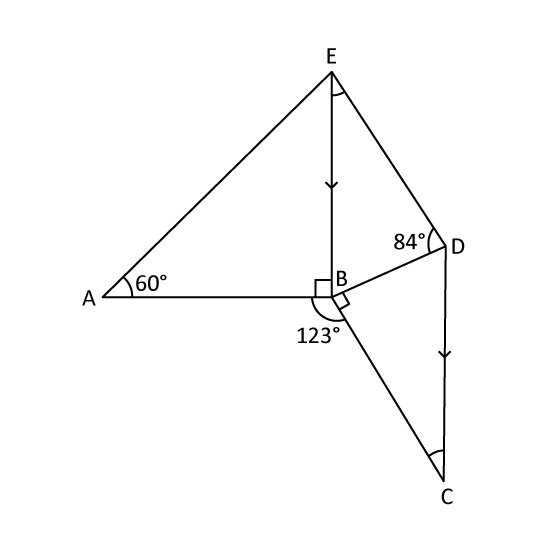Level 1 PSLE
Robert cut out three identical right-angled triangles. He joined them to form the figure PQRS shown. RS = 12 cm and SP = 10 cm. The perimeter of the figure is 36 cm. Find the area of the figure PQRS.1 m
Level 2
The figures X and Y, are two identical isosceles triangles. Both figures contain a square of a different size. Given that the area of the square in Figure Y is 360 cm2, find the area of the square in Figure X.2 m
Level 2 PSLE
Anna has a square piece of paper FGHJ of side 21 cm. She cut along the dotted lines shown in Figure 1 to get one small square of area 9 cm2 and 8 identical right-angled triangles. Triangle KLM in Figure 2 is one such triangle. Find the length of KM.2 m
Level 2 PSLE
In the figure, ABDF and BCEF are rectangles and CDE is a straight line. AB = 6 cm, AF = 8 cm and BF = 10 cm. Find the length of BC.2 m
Level 2 PSLE
In the figure, MN = 7 cm, NO = 9 cm, OP = 3 cm and PM = 11 cm. ∠MNO and ∠OPM are right angles. Find the area of the figure MNOP.2 m
Level 2
The shaded figure is made up of 4 quarter arcs of radius 12 cm. Find its area. (Take π = 3.14)2 m
Level 2
The figure is formed by a circle and an isosceles triangle where PQ = PR. The radius of the circle is 14 cm. Find the area of the shaded part. (Take π = 227)2 m
Level 3
In the figure, ABE and DBC are right-angled triangles. EB is parallel to DC. Find
1. ∠BCD
2. ∠BED3 m
Level 3
The figure is made up of a right-angled isosceles triangle XYZ and a semicircle. XY = YZ and the diameter of the semicircle is 28 cm. Find the area of the shaded part of the figure.3 m
Level 3
Two identical right-angled triangles overlap each other as shown. Find the area of the shaded part.2 m